# Maxima and Minima

#### Topics

• First and Second Derivative test
• Determine critical points of the function
• Find the point(s) of local maxima and local minima and corresponding local maximum and local minimum values
• Find the absolute maximum and absolute minimum value of a function

## Definition

Let f be a function defined on an interval I. Then

(a) f is said to have a maximum value in I, if there exists a point c in I such that f(c )>f (x),  for all x ∈ I.
The number f(c) is called the maximum value of f in I and the point c is called a point of maximum value of f in I.

(b) f  is said to have a minimum value in I, if there exists a point c in I such that f(c) < f(x), for all x ∈ I.
The number f(c), in this case, is called the minimum value of f in I and the point c, in this case, is called a point of minimum value of  f  in I.

(c) f is said to have an extreme value in I if there exists a point c in I such that f (c) is either a maximum value or a minimum value of f  in I.
The number  f(c), in this case, is called an extreme value of  f  in I and the point c is called an extreme point.

Remark:  In  following Fig.  the graphs of certain particular functions  help us to find maximum value and minimum value at a point. Infact, through graphs, we can even find maximum/minimum value of a function at a point at which it is not even differentiable.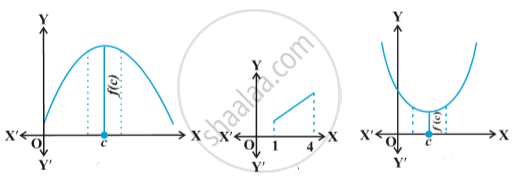## Definition

Let  f  be a real valued function and let c be an interior point in the domain of f. Then

(a) c is called a point of local maxima if there is an h > 0 such that
f(c) ≥ f(x), for all x in (c – h, c + h), x ≠ c
The value f(c) is called the local maximum value of f.

(b) c is called a point of local minima if there is an h > 0 such that
f(c) ≤ f(x), for all  x in (c – h, c + h)

The value f(c) is called the local minimum value of f.

Geometrically, the above definition states that if  x = c is a point of local maxima of  f, then the graph of f around c will be as shown in Fig. Note that the function f  is increasing (i.e., f′(x) > 0) in the interval (c – h, c) and decreasing (i.e., f′(x) < 0) in the interval (c, c + h).
This suggests that f′(c) must be zero.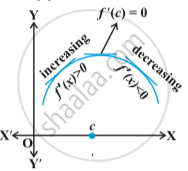Similarly, if c is a point of local minima of f , then the graph of f around c will be as shown in following Fig. Here f  is decreasing (i.e., f′(x) < 0) in the interval (c – h, c) and increasing (i.e., f′(x) > 0) in the interval (c, c + h). This again suggest that f′(c) must be zero.
The above discussion lead us to the following theorem (without proof).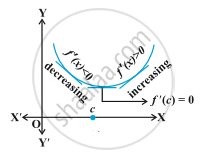## Theorem

Let  f  be a function defined on an open interval I. Suppose c ∈ I be  any point. If f  has a local maxima or a local minima at x = c, then either f′(c) = 0 or f  is not differentiable at c.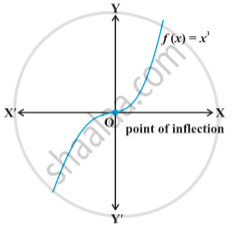Remark: The converse of above theorem need not be true, that is, a point at which the derivative vanishes need not be a point of local maxima or local minima. For example, if f(x) = x^3, then f′(x) = 3x^2 and so f′(0) = 0. But 0 is neither a point of local maxima nor a point of local minima  in above Fig.

## Theorem

(First Derivative Test):
Let f be a function defined on an open interval I. Let f be continuous at a critical point c in I. Then

(i) If f′(x) changes sign from positive to negative as x increases through c, i.e., if f′(x) > 0 at every point sufficiently close to and to the left of c, and f′(x) < 0 at every point sufficiently close to and to the right of c, then c is a point of local maxima.

(ii) If f′(x) changes sign from negative to positive as x increases through c, i.e., if f′(x) < 0 at every point sufficiently close to and to the left of c, and f′(x) > 0 at every point sufficiently close to and to the right of c, then c is a point of local minima.

(iii) If f′(x) does not change sign as x increases through c, then c is neither a point of local maxima nor a point of local minima. Infact, such a point is called point of inflection Fig.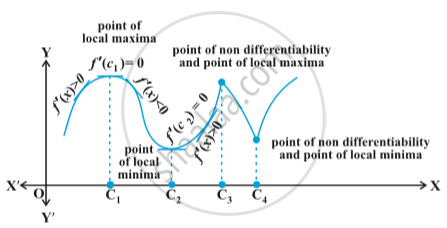## Theorem

Second Derivative Test:
Let  f  be a function defined on an interval I and c ∈ I. Let f  be twice differentiable at c. Then

(i) x = c is a point of local maxima if f′(c) = 0 and f″(c) < 0 The value f (c) is local maximum value of  f .

(ii) x = c is a point of local minima if f'(c) =  and f″(c) > 0 In this case, f (c) is local minimum value of f.

(iii) The test fails if f′(c) = 0 and f″(c) = 0. In this case, we go back to the first derivative test and find whether c is a point of local maxima, local minima or a point of inflexion.

If you would like to contribute notes or other learning material, please submit them using the button below.

### Shaalaa.com

Application of Derivatives part 21 (First Derivative Test) [00:07:53]
S
0%